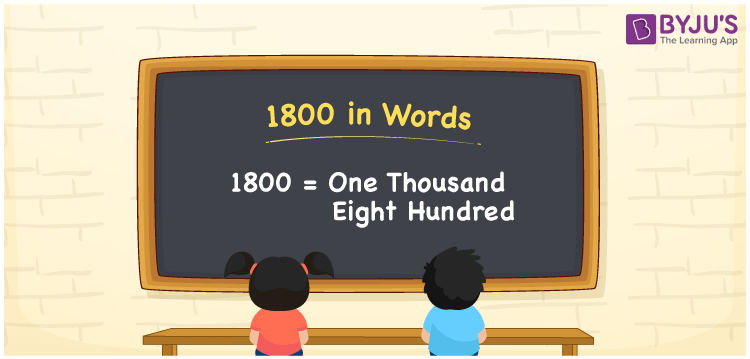# 1800 in words

1800 in words is written as One Thousand Eight Hundred. 1800 represents the count or value. The article on Counting Numbers can give you an idea about count or counting. The number 1800 is used in expressions that relate to money, distance, length and others. Let us consider an example for 1800. “The famous singer has sung One Thousand Eight Hundred songs” .

 1800 in words One Thousand Eight Hundred One Thousand Eight Hundred in Numbers 1800

## 1800 in English Words## How to Write 1800 in Words?

We can convert 1800 to words using a place value chart. The number 1800 has 4 digits, so let’s make a chart that shows the place value up to 4 digits.

 Thousands Hundreds Tens Ones 1 8 0 0

Thus, we can write the expanded form as:

1 × Thousand + 8 × Hundred + 0 × Ten + 0 × One

= 1 × 1000 + 8 × 100 + 0 × 10 + 0 × 1

= 1800

= One Thousand Eight Hundred.

1800 is the natural number that is succeeded by 1799 and preceded by 1801.

1800 in words – One Thousand Eight Hundred.

Is 1800 an odd number? – No.

Is 1800 an even number? – Yes.

Is 1800 a perfect square number? – No.

Is 1800 a perfect cube number? – No.

Is 1800 a prime number? – No.

Is 1800 a composite number? – Yes.

## Solved Example

1. Write the number 1800 in expanded form

Solution: 1 × 1000 + 8 × 100 + 0 × 10 + 0 × 1

We can write 1800 = 1000 + 800 + 0 + 0

= 1 × 1000 + 8 × 100 + 0 × 10 + 0 × 1

## Frequently Asked Questions on 1800 in words

Q1

### How to write 1800 in words?

1800 in words is written as One Thousand Eight Hundred.
Q2

### State True or False. 1800 is divisible by 2?

True. 1800 is divisible by 2.
Q3

### Is 1800 divisible by 10?

Yes. 1800 is divisible by 10. It is also divisible by 2, 4, 5, 10 and 100.